## 5-2 Volume (嚙踝蕭嚙踝蕭嚙踝蕭)

[chinese][all]

The loudness of audio signals is the most prominent features according to human aural perception. In general, there are several interchangeable terms that are commonly used to describe the loudness of audio signals, including volume, energy, and intensity. For consistency, here we shall use the term "volume" for describing the loudness. Basically, volume is an acoustic feature that is correlated to the samples' amplitudes within a frame. To define volume quantitatively, we can employ two methods to compute the volume of a given frame:

1. The sum of absolute samples within each frame: $$volume = \sum_{i=1}^n |s_i|$$ where $s_i$ is the $i$-th sample within a frame, and $n$ is the frame size. This method requires only integer operations and it is suitable for low-end platforms such as micro-controllers.
2. 10 times the 10-based logarithm of the sum of sample squares: $$volume = 10*log_{10} \sum_{i=1}^n s_i^2$$ This method requires floating-point computations, but it is (more or less) linearly correlated to our perception of loudness of audio signals. The quantity computed is also referred as the "log energy" in the unit of decibels. More explanations about decibels can be found in the following page:
http://www.phys.unsw.edu.au/~jw/dB.html (local copy)

Some characteristics of volume are summarized next.

• For recording in a common quiet office using a uni-directional microphone, the volume of voiced sounds is usually larger than that of unvoiced sounds, and the volume of unvoiced sounds is usually larger than that of environmental noise. (However, this is not applicable to recordings via the omni-directional microphone.)
• Volume is greatly influenced by microphone setups, mostly the microphone gain.
• Volume is usually used for endpoint detection which tries to find the region of meaningful voice activity.
• Before computing the volume, we usually perform zero-justification (by simply subtracting the mean or median of the frame from each samples) to avoid potential DC-bias, as follows.
• For method of "abs sum", we usually apply median subtraction for zero justification.
• For method of "log squared sum", we usually apply mean subtraction for zero justification.

The following example demonstrates how to use these two methods for volume computation:

Example 1: volume01.mwaveFile='sunday.wav'; frameSize=256; overlap=128; au=myAudioRead(waveFile); y=au.signal; fs=au.fs; fprintf('Length of %s is %g sec.\n', waveFile, length(y)/fs); frameMat=enframe(y, frameSize, overlap); frameNum=size(frameMat, 2); % Compute volume using method 1 volume1=zeros(frameNum, 1); for i=1:frameNum frame=frameMat(:,i); frame=frame-median(frame); % zero-justified volume1(i)=sum(abs(frame)); % method 1 end % Compute volume using method 2 volume2=zeros(frameNum, 1); for i=1:frameNum frame=frameMat(:,i); frame=frame-mean(frame); % zero-justified volume2(i)=10*log10(sum(frame.^2)+realmin); % method 2 end sampleTime=(1:length(y))/fs; frameTime=((0:frameNum-1)*(frameSize-overlap)+0.5*frameSize)/fs; subplot(3,1,1); plot(sampleTime, y); ylabel(waveFile); subplot(3,1,2); plot(frameTime, volume1, '.-'); ylabel('Volume (Abs. sum)'); subplot(3,1,3); plot(frameTime, volume2, '.-'); ylabel('Volume (Decibels)'); xlabel('Time (sec)');Length of sunday.wav is 0.98225 sec.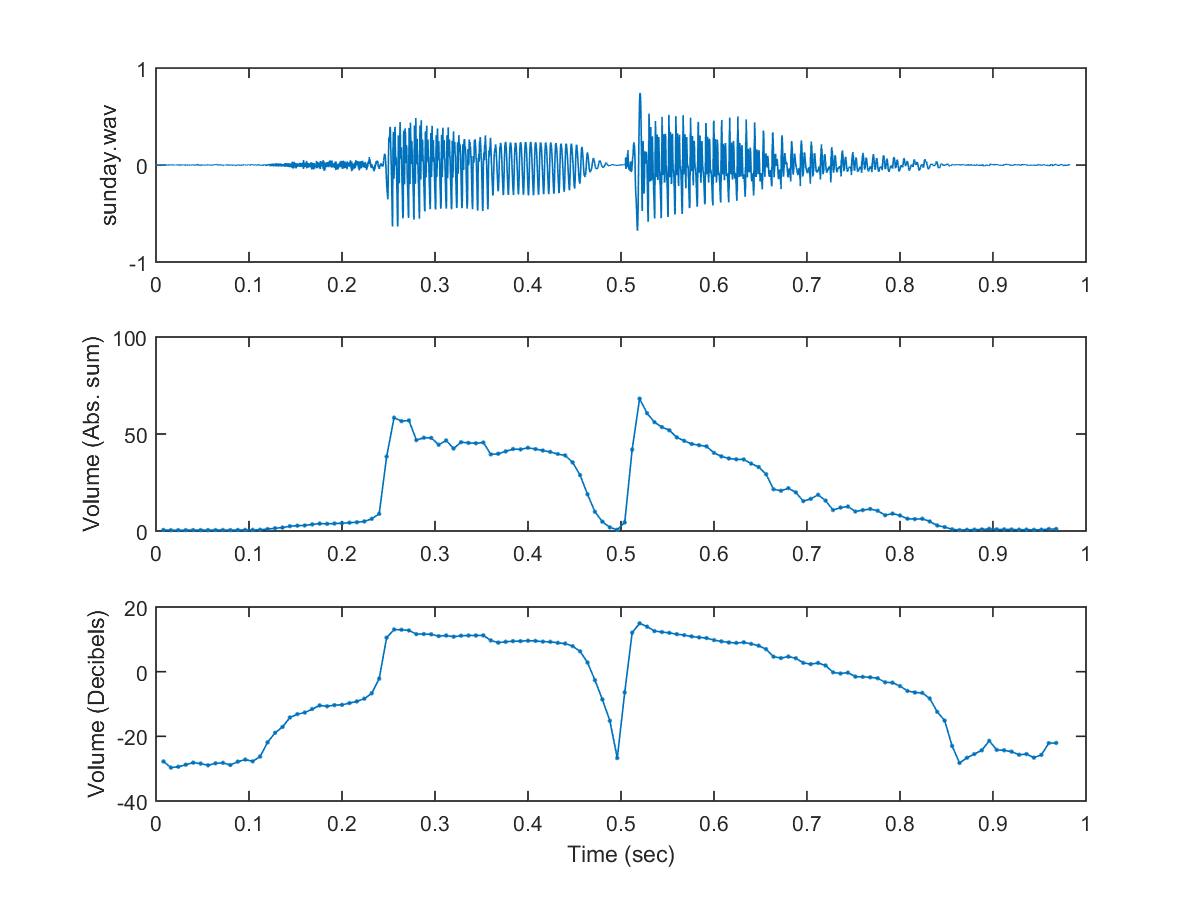Since we need to compute the volume in many different applications, so we have created a function "frame2volume" (in SAP toolbox) which can compute the volume from a given frame. So we can use the function to simply the previous example:

Example 2: volume02.mwaveFile='sunday.wav'; frameSize=256; overlap=128; au=myAudioRead(waveFile); y=au.signal; fs=au.fs; fprintf('Length of %s is %g sec.\n', waveFile, length(y)/fs); frameMat=enframe(y, frameSize, overlap); frameNum=size(frameMat, 2); opt=frame2volume('defaultOpt'); opt.method='absSum'; volume1=frame2volume(frameMat, opt); opt.method='decibel'; volume2=frame2volume(frameMat, opt); sampleTime=(1:length(y))/fs; frameTime=frame2sampleIndex(1:frameNum, frameSize, overlap)/fs; subplot(3,1,1); plot(sampleTime, y); ylabel(waveFile); subplot(3,1,2); plot(frameTime, volume1, '.-'); ylabel('Volume (Abs. sum)'); subplot(3,1,3); plot(frameTime, volume2, '.-'); ylabel('Volume (Decibels)'); xlabel('Time (sec)');Length of sunday.wav is 0.98225 sec.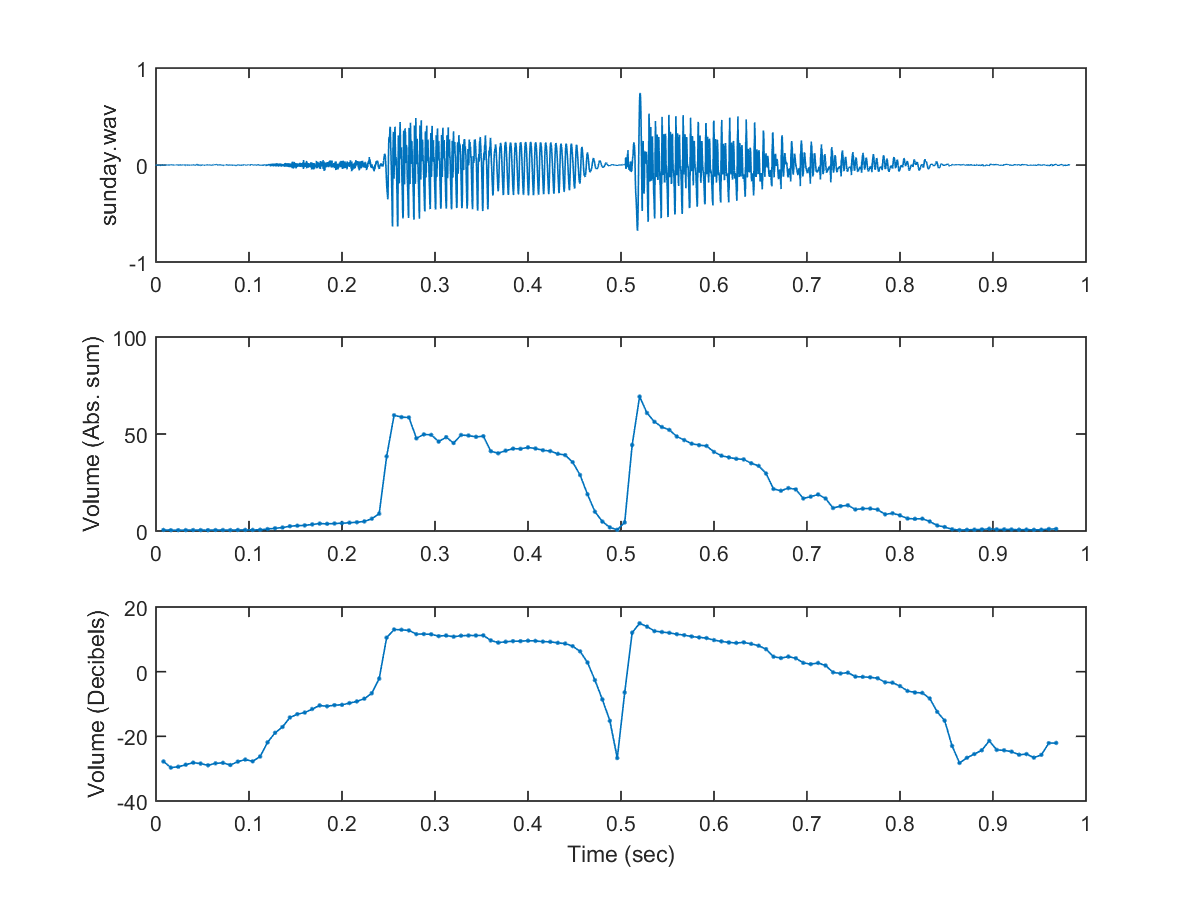To go one step further, we can use the function "wave2volume" (in SAP toolbox) which computes volume from a given wave file:

Example 3: volume03.mwaveFile='sunday.wav'; au=myAudioRead(waveFile); opt=wave2volume('defaultOpt'); opt.frameSize=256; opt.overlap=128; time=(1:length(au.signal))/au.fs; subplot(3,1,1); plot(time, au.signal); xlabel('Time (sec)'); ylabel('Amplitude'); title(waveFile); subplot(3,1,2); opt1=opt; opt1.frame2volumeOpt.method='absSum'; vollume1=wave2volume(au, opt1, 1); ylabel(opt1.frame2volumeOpt.method); subplot(3,1,3); opt2=opt; opt2.frame2volumeOpt.method='decibel'; volume2=wave2volume(au, opt2, 1); ylabel(opt2.frame2volumeOpt.method);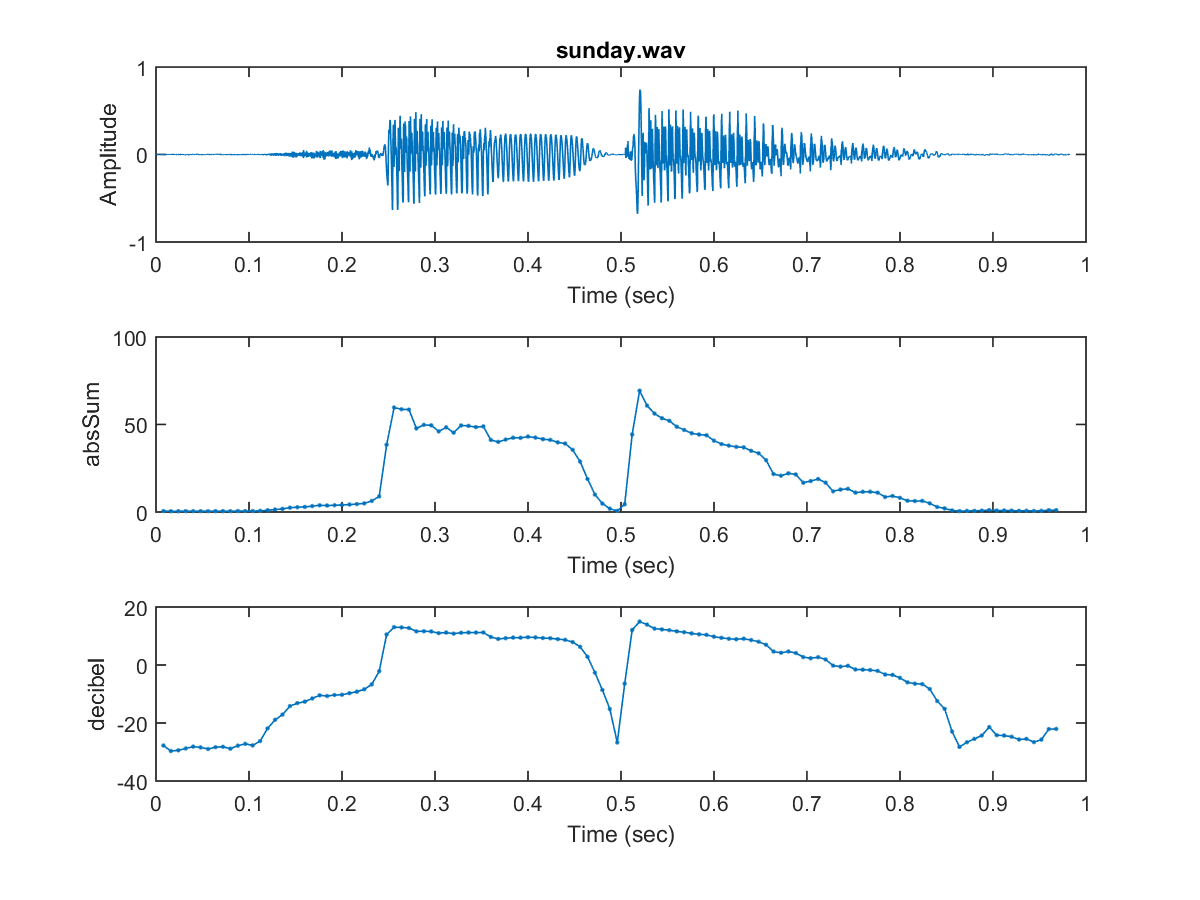The above two methods of computing volume are only an approximation to our perception of loudness. However, the loudness is based on our perception and there could be significant differences between the "computed loudness" and the "perceived loudness". In fact, the perceived loudness is greatly affected by the freqency as well as the timber of the audio signals. If we plot the equal perceived loudness against sinusoidal signals of varied frequencies, we will have the following curves of equal loudness: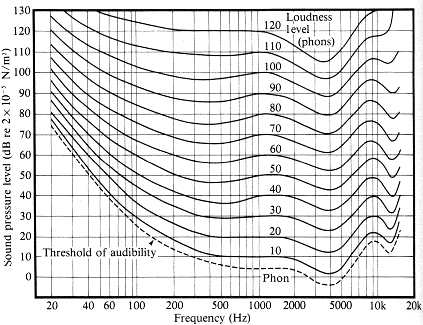Curves of equal loudness determined experimentally by Fletcher, H. and Munson, W.A. (1933) J.Acoust.Soc.Am. 6:59.

The above figure also shows the sensitivity of the human ear with respect to frequency, which is simply the frequency response of the human ear. If you want to obtain the frequency response of your ears, you can jump to the the "Equal Loudness Tester" pages:

http://www.phys.unsw.edu.au/~jw/hearing.html (local copy)

Besides frequencies, the perceived loudness is also influenced by the the frame's timbre. For instance, we can try to pronounce several vowels using the same loudness level, and then plot the volume curves to see how they are related to the timbre or shapes/positions of lips/tongue, as shown in the following example.

Example 4: volume04.mwaveFile='aeiou.wav'; au=myAudioRead(waveFile); opt=wave2volume('defaultOpt'); opt.frameSize=512; opt.overlap=0; time=(1:length(au.signal))/au.fs; subplot(3,1,1); plot(time, au.signal); xlabel('Time (sec)'); ylabel('Amplitude'); title(waveFile); subplot(3,1,2); opt1=opt; opt1.frame2volumeOpt.method='absSum'; vollume1=wave2volume(au, opt1, 1); ylabel(opt1.frame2volumeOpt.method); subplot(3,1,3); opt2=opt; opt2.frame2volumeOpt.method='decibel'; volume2=wave2volume(au, opt2, 1); ylabel(opt2.frame2volumeOpt.method);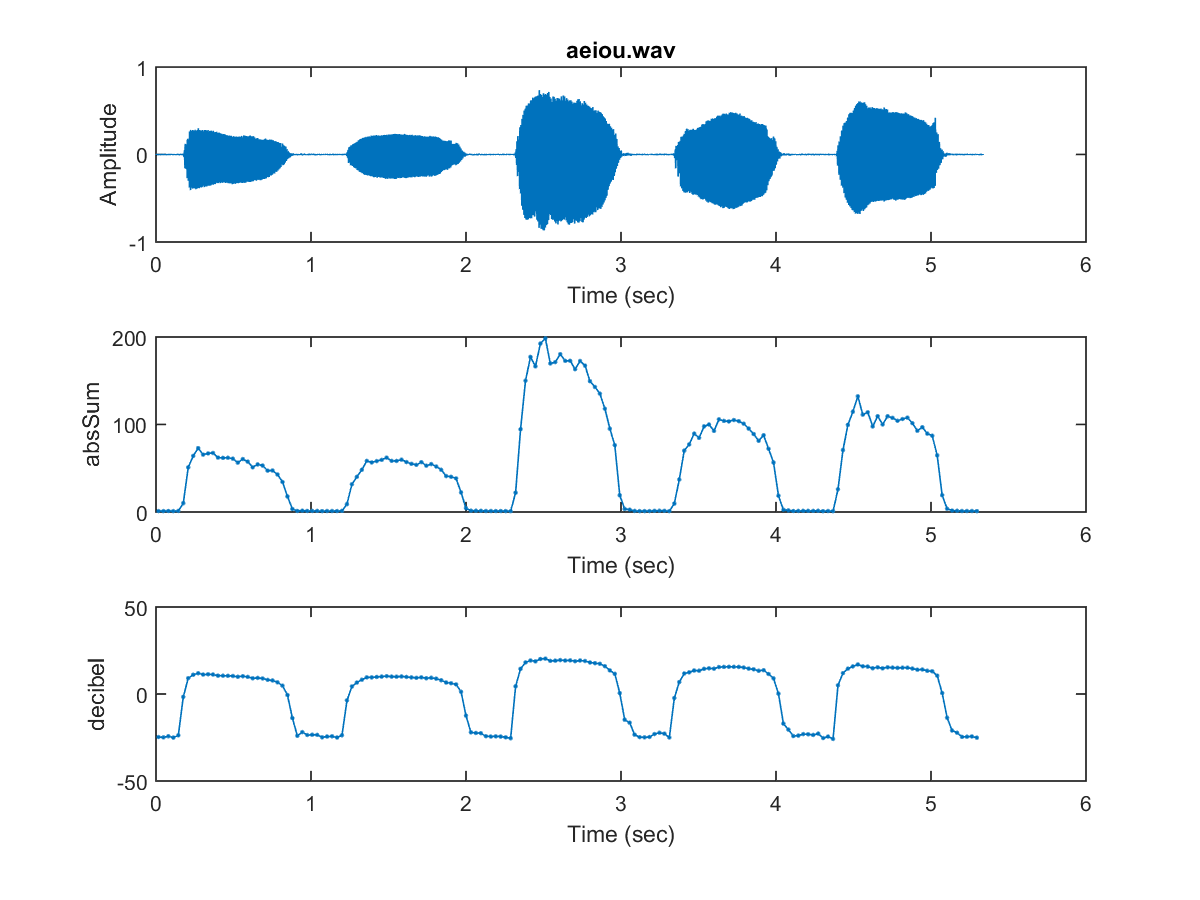From the above example, you can observed that though the perceived loudness is the same (click here to hear the recording), the computed volumes depend a lot on the timbers. In fact, we can perform another experiment to pronounce the same vowel but with different pitch to see how the perceived loudness depends on the fundamental frequency. This is left as an exercise.

Since the perceived loudness is easily affected by the fundamental frequency as well as the timber, we need to adjust the amplitudes accordingly when we are performing text-to-speech synthesis or singing voice synthesis.

Audio Signal Processing and Recognition (音訊處理與辨識)